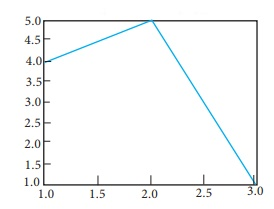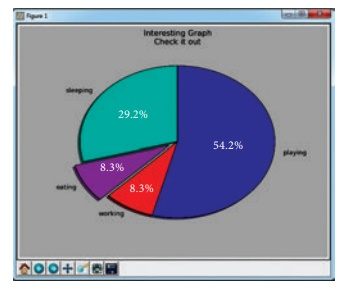Home | | Computer Science 12th Std | Data Visualization Using Pyplot: Book Back Questions and Answers

# Data Visualization Using Pyplot: Book Back Questions and Answers

Evaluation

Part – I

Choose the best answer (1 Marks)

1.Which is a python package used for 2D graphics?

a. matplotlib.pyplot

b. matplotlib.pip

c. matplotlib.numpy

d. matplotlib.plt

2.Identify the package manager for Python packages, or modules.

a. Matplotlib

b. PIP

c. plt.show()

d. python package

3.Read the following code: Identify the purpose of this code and choose the right option from the following.

C:\Users\YourName\AppData\Local\Programs\Python\Python36-32\Scripts>pip – version

a. Check if PIP is Installed

b. Install PIP

d. Check PIP version

4.Read the following code: Identify the purpose of this code and choose the right option from the following.

a. List installed packages

b. list command

c. Install PIP

d. packages installed

5.To install matplotlib, the following function will be typed in your command prompt. What does “-U”represents?

Python –m pip install –U pip

c. removing pip

6. Observe the output figure. Identify the coding for obtaining this output.a. import matplotlib.pyplot as plt

plt.plot([1,2,3],[4,5,1])

plt.show()

b. import matplotlib.pyplot as plt

plt.plot([1,2],[4,5])

plt.show()

c. import matplotlib.pyplot as plt

plt.plot([2,3],[5,1])

plt.show()

d. import matplotlib.pyplot as plt

plt.plot([1,3],[4,1])

plt.show()

a. import matplotlib.pyplot as plt

b. plt.plot(3,2)

c. plt.show()

Identify the output for the above coding.8.Which key is used to run the module?

a. F6

b. F4

c. F3

d. F5

9.Identify the right type of chart using the following hints.

Hint 1: This chart is often used to visualize a trend in data over intervals of time.

Hint 2: The line in this type of chart is often drawn chronologically.

a.  Line chart

b. Bar chart

c. Pie chart

d. Scatter plot

10. Read the statements given below. Identify the right option from the following for pie chart.

Statement A: To make a pie chart with Matplotlib, we can use the plt.pie() function.

Statement B: The autopct parameter allows us to display the percentage value using the Python string formatting.

a. Statement A is correct

b. Statement B is correct

c. Both the statements are correct

d. Both the statements are wrong

Part - II

Answer the following questions       (2 Marks)

1. Define: Data Visualization.

Ans. Data Visualization is the graphical representation of information and data. The objective of Data Visualization is to communicate information visually to users. For this, data visualization uses statistical graphics. Numerical data may be encoded using dots, lines, or bars, to visually communicate a quantitative message.

2. List the general types of data visualization.

Ans. (i) Charts

(ii) Tables

(iii) Graphs

(iv) Maps

(v) Infographics

(vi) Dashboards

3. List the types of Visualizations in Matplotlib.

Ans. There are many types of Visualizations under Matplotlib. Some of them are:

(i) Line plot

(ii) Scatter plot

(iii) Histogram

(iv) Box plot

(v) Bar chart and

(vi) Pie chart

4. How will you install Matplotlib?

Ans. You can install the latest version of pip from your command prompt using the following command.

Python-m pip install -U pip

5. Write the difference between the following functions: plt.plot([1,2,3,4]), plt. plot([1,2,3,4], [1,4,9,16]).

Ans.plt.plot([l,2,3,4])

After installing Matplotlib, we will begin coding by importing Matplotlib using the command: import matplotlib. pyplot as pIt

Now you have imported Matplotlib in your workspace.

You need to display the plots. Using Matplotlib from within a Python script, you have to add plt.show() method inside the file to display your plot.

plt.plot([l,2,3,4], [1,4,9,16])

A single list or array provided to the plot () command, matplotlib assumes it is a sequence of y values, and automatically generates the x values for you. Since python ranges start with 0, the default x vector has the same length as y but starts with 0.

Hence the x data are [0, 1,2,3].

plot() is a versatile command, and will take an arbitrary number of arguments.

This .plot takes many parameters, but the first two here are 'x' and 'y' coordinates. This means, you have 4 co-ordinates according to these lists: (1,1), (2,4), (3,9) and (4,16).

Part – III

Answer the following questions (3 Marks)

1. Draw the output for the following data visualization plot.

import matplotlib.pyplot as plt

plt.bar([1,3,5,7,9],[5,2,7,8,2], label="Example one")

plt.bar([2,4,6,8,10],[8,6,2,5,6], label="Example two", color='g')

plt.legend()

plt.xlabel('bar number')

plt.ylabel('bar height')

plt.title('Epic Graph\nAnother Line! Whoa')

plt.show()

Ans.The above code represents the following

Lables → specifics labels for the bars.

Usage → Assign values to the labels specified.

Range → create sequence of numbers.

2. Write any three uses of data visualization.

Ans. (i) Data Visualization help users to analyze and interpret the data easily.

(ii) It makes complex data understandable and usable.

(iii) Various Charts in Data Visualization helps to show relationship in the data for one or more variables.

3. Write the coding for the following:

a. To check if PIP is Installed in your PC.

b. To Check the version of PIP installed in your PC.

c. To list the packages in matplotlib.

Ans. a. pip

b. pip—version

c. pip list

4. Write the plot for the following pie chart output.Ans. import matplotlib.pyplot as pit

val = [29.2, 54.2, 6.3, 8.35]

label = ["sleeping", "playing", "working", eating"]

plt.pie (val, label = label)

plt.axes (). set_aspect ("equal")

plt.title ("Interesting Graph Check it out")

plt.show()

Part – IV

Answer the following questions (5 Marks)

1. Explain in detail the types of pyplots using Matplotlib.

Ans. Matplotlib allows you to create different kinds of plots ranging from histograms and scatter plots to bar graphs and bar charts.

Line Chart:

(i) A Line Chart or Line Graph is a type of chart which displays information as a series of data points called ‘markers’ connected by straight line segments.

(ii) A Line Chart is often used to visualize a trend in data over intervals of time - a time series - thus the line is often drawn chronologically.

(iii) Program for Line plot:

import matplotlib.pyplot as pIt

years = [2014, 2015, 2016, 2017, 2018]

total_populations = [8939007, 8954518, 8960387,8956741, 8943721]

plt.plot (years, total_populations)

plt.title ("Year vs Population in India")

plt.xlabel ("Year")

plt.ylabel ("Total Population")

plt.show()

In this program,

Plt.title() → specifies title to the graph

Plt.xlabelO → specifies label for X-axis

Plt.ylabel() → specifies label for Y-axis

OutputBar Chart:

(i) A BarPlot (or BarChart) is one of the most common type of plot. It shows the relationship between a numerical variable and a categorical variable.

(ii) Bar chart represents categorical data with rectangular bars. Each bar has a height corresponds to the value it represents. The bars can be plotted vertically or horizontally.

(iii) It’s useful when we want to compare a given numeric value on different categories. To make a bar chart with Matplotlib, we can use the plt.bar() function.

(iv) Program :

import matplotlib.pyplot as pit

#Our data

labels = ["TAMIL", "ENGLISH", "MATHS", "PHYSICS", "CHEMISTRY", "CS"]

usage = [79.8, 67.3, 77.8, 68.4, 70.2, 88.5]

#Generating the y positions. Later, we'll use them to replace them with labels.

y_positions = range (len(labels))

# Creating our bar plot

plt.bar (y_positions, usage)

plt.xticks (y_positions, labels)

plt.ylabel ("RANGE")

plt.title ("MARKS")

plt.show()

Output:Pie Chart:

(i) Pie Chart is probably one of the most common type of chart. It is a circular graphic which is divided into slices to illustrate numerical proportion.

(ii) The point of a pie chart is to show the relationship of parts out of a whole. To make a Pie Chart with Matplotlib, we can use the plt.pie() function.

(iii) The autopct parameter allows us to display the percentage value using the Python string formatting.

(iv) Program:

import matplotlib.pyplot as pit

sizes = [89, 80, 90,100, 75]

labels = ["Tamil", "English", "Maths", "Science", "Social"]

plt.pie (sizes, labels = labels, autopct = "%2f")

plt.axes().set_aspect ("equal")

plt.show()

Output:2. Explain the various buttons in a matplotlib window.

Ans.

Buttons in the output : In the output figure, you can see few buttons at the bottom left corner. Let us see the use of these buttons.(i) Home Button → The Home Button will help one to begun navigating the chart. If you ever want to return back to the original view, you can click on this.

(ii) Forward/Back buttons → These buttons can be used like the Forward and Back buttons in browser. Click these to move back to the previous point you were at, or forward again.

(iii) Pan Axis → This cross-looking button allows you to click it, and then click and drag graph around.

(iv) Zoom → The Zoom button lets you click on it, then click and drag a square would like to zoom into specifically. Zooming in will require a left click and drag. Zoom out with a right click and drag.

(v) Configure Subplots → This button allows you to configure various spacing options with figure and plot.

3. Explain the purpose of the following functions:

a. plt.xlabel

b. plt.ylabel

c. plt.title

d. plt.legend()

e. plt.show()

Ans. a. Specifies label for x-axis

b. Specifies label for y-axis

c. Specifies title to the graph or assigns the plot title.

d. Invoke the default legend with pit

e. Display the plot

Tags : Line Chart, Pie Chart and Bar Chart , 12th Computer Science : UNIT 16 : Integrating Python with MySql and C++ : Data Visualization Using Pyplot: Line Chart, Pie Chart and Bar Chart
Study Material, Lecturing Notes, Assignment, Reference, Wiki description explanation, brief detail
12th Computer Science : UNIT 16 : Integrating Python with MySql and C++ : Data Visualization Using Pyplot: Line Chart, Pie Chart and Bar Chart : Data Visualization Using Pyplot: Book Back Questions and Answers | Line Chart, Pie Chart and Bar Chart